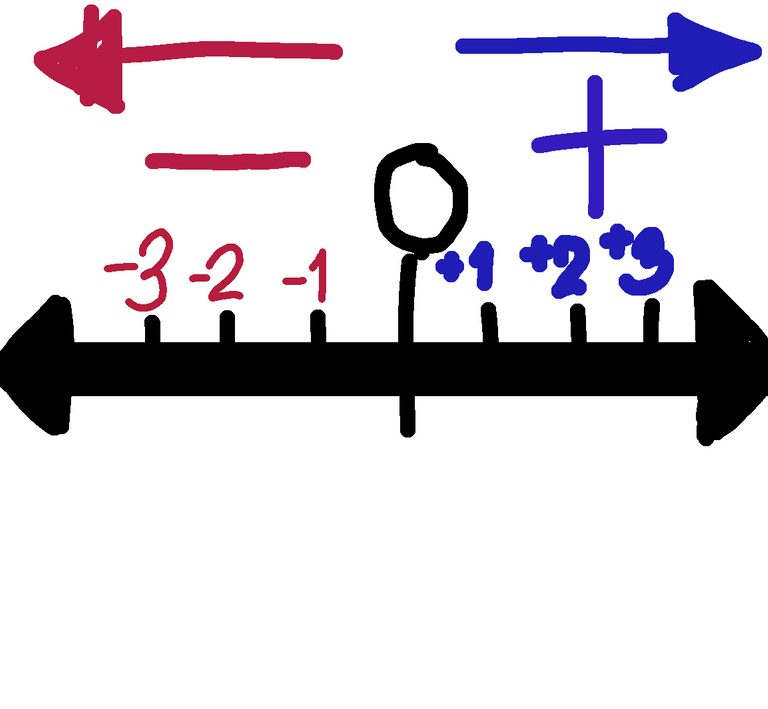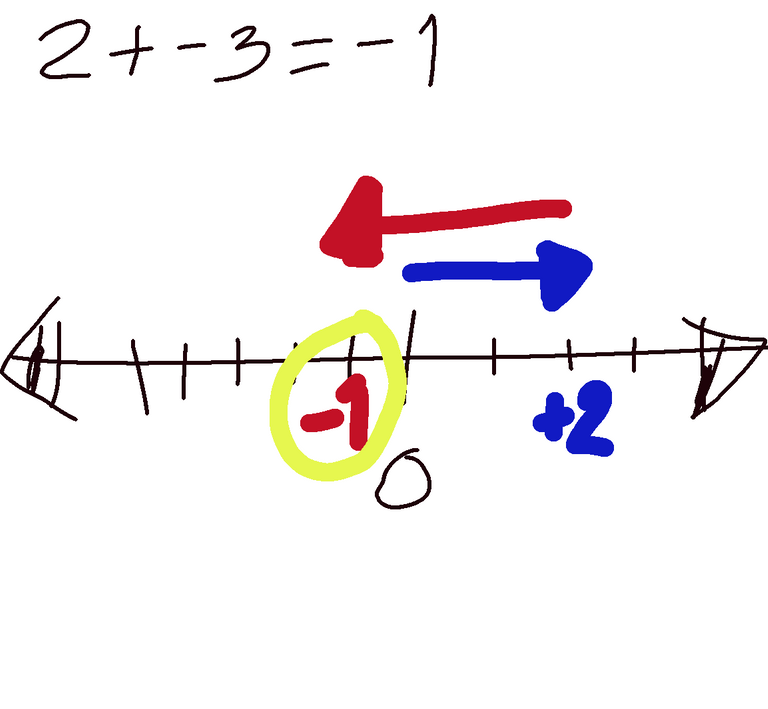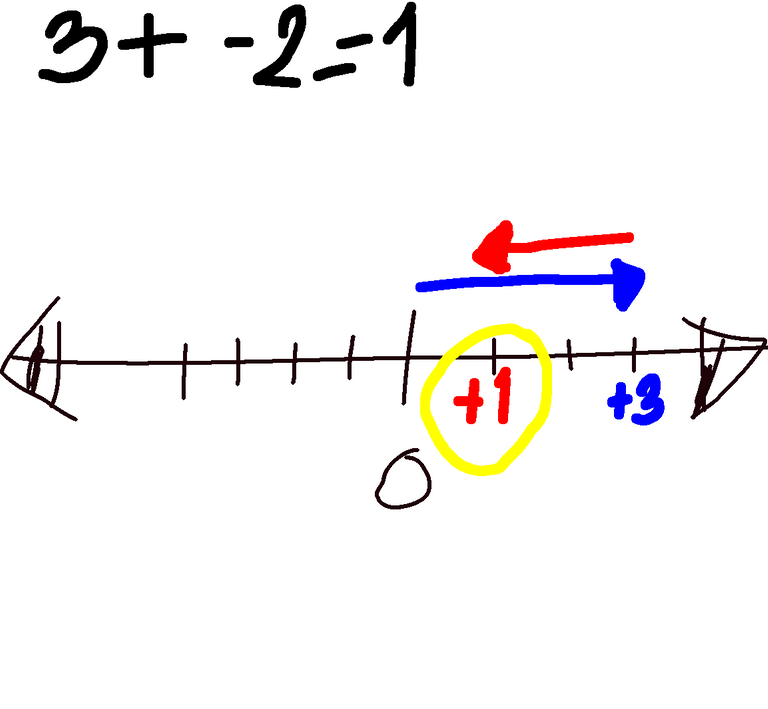in Educationlast year

Math is one of the most feared subject for some i do agree to it since it is so abstract. But how about we make it easier. Let me start with the Addition of Integers

Integers as for me are numbers with sign attached to them like - negative and + positive except zero, but 0 is an integer too.Numbers to the right if you are facing the phone and the colored blue are the positive integers. While the numbers to the left if you are facing the phone and the colored red are the negative integers. All have their on signs attached to them as per definition but if you are advance you can just write positive integers without having their signs.

How do we utilize the use of the number line. Take a look at some examples.

1. 2 + -3= -1

Why?Observe:
Numbers with positive sign will move to the right as you can see in the image above. The movement will start from 0 for the first movement. Since we started with 2 we move to the right twice stopping at +2. Now the second movement will start from where the first movement ended. Since our first movement ended at +2 we will start moving from positive 2 going to the left since the negative sign will tell you to move going to the left and we will move by 3 since it is negative 3. As the result we stop at -1 and that is our final destination for this example so we write -1 as the answer.

Advance: 2+ -3= -1, since they got different sign and in addition operation just subtract it and copy the sign of the biggest number. From the example the difference of 2 and 3 is 1 and the biggest number is 3 which is negative therefore the sign of our answer must be negative thus our answer is -1.

Note: You can choose where to start just remember that the second movement will always start at the end of the first movement.

Example 2: 3+-2=1
Why?Again positive numbers will always move to the right like what is shown above. Since it is +3 we move to the right 3 times starting from 0 and it will stop at +3 the second movement will then start from where the first movement ended which is at +3 and it will go left since it is -2 and we move to the left twice ending at +1 which will then be our answer.

Advance: Since they have different sign and in addition operation we will just subtract them and copy the sign of the biggest number. Since the difference between 2 and 3 is 1 and the biggest number is positive therefore our answer is +1.

Try this:

1. 5+-6=
2. 7+-1=
3. 9+-10=
4. -11+9=
5. -3+2=

I want to check if you followed my discussion and i will be expecting your answers and suggestions below in the comment section.

Sort:

When teaching addition and subtraction of positive and negatives, I make use of a little bird image and depending on the operation, and +/- value of each number in the equation, the bird will face different directions. It doesn't sound very helpful here, but when explained correctly in class, I find this process really assists students in applying this concept.

Somehow it is another approach to teaching abstract topic like math having the concrete example. As they say there are many ways on how to kill a chicken. Thank you for sharing your strat..

Your content has been voted as a part of Encouragement program. Keep up the good work!

Use Ecency daily to boost your growth on platform!

Support Ecency
Vote for Proposal
Delegate HP and earn more